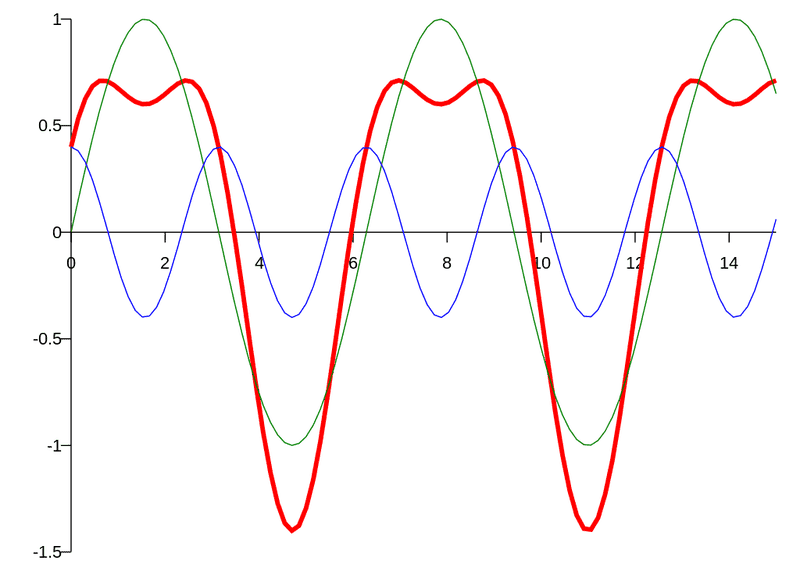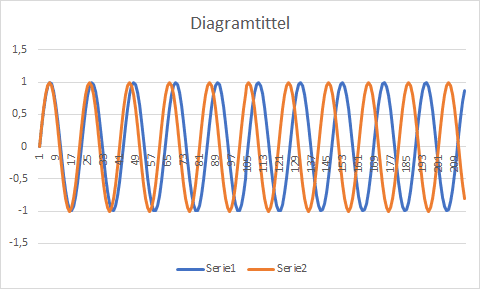# Can a function have two fundamental periods?

• B
jaumzaum
I was wondering if a periodic function could have 2 different fundamental periods? If so, could you give an example? And If not, how can I prove that?

Mentor
I was wondering if a periodic function could have 2 different fundamental periods? If so, could you give an example? And If not, how can I prove that?
I don't see how it could. The sine function has a period of ##2\pi##, since ##\sin(x) = \sin(x + 2\pi)##, for any real x. It's also true that ##\sin(x) = \sin(x + k \cdot 2\pi)## for any positive integer k, but unless I'm mistaken, the fundamental period is the smallest number p for which ##\sin(x) = \sin(x + p)##.

••WWGD, jaumzaum, mcastillo356 and 1 other person
Homework Helper
Gold Member
If there are two frequencies in question, then either their periods will never match up (e.g. one has period 1 and the other has period ##\pi##), or they would match up at some time, ##t_{period}##. In the second case, you would want to define the fundamental period as ##t_{period}## rather than the individual smaller periods. To appreciate the reason for that, consider the saw-tooth wave. You would say that its period is the period of the saw-tooth, but it is made up of many frequencies with shorter periods. None of those faster frequencies gives a fundamental period for the saw-tooth.
CORRECTION: The above is all wrong. As others have pointed out, the fundamental period is the smallest period, so there can not be two of them.

Last edited:
•jaumzaum and DaveE
Staff Emeritus
Gold Member
The fundamental period is usually defined as the smallest period, which is unique. If it exists. It might not, for example ##f(x)## defined to be 1 if ##x\in \mathbb{Q}## and 0 otherwise has every rational number as a period, and no fundamental period.

This might feel a little disappointing - given two periods there's still a single smaller period that generates both of them. Consider instead two real numbers ##x_1## and ##x_2## that are not rational multiples of each other. Then it turns out integer combinations of them get arbitrarily small. So ##f(x)## defined to be 1 if ##x=mx_1 + nx_2## for some integers m and n, and 0 otherwise, has as periods every integer combination of ##x_1## and ##x_2##, hence no fundamental period. But you can't find a single smaller period that makes the existence of ##x_1## and ##x_2## as periods trivial, i.e. there isn't some ##p## such that ##mp=x_1## and ##np=x_2##.

Last edited:
•jaumzaum
jaumzaum
Sorry by that misunderstanding. What I meant by two different fundamental periods was two periods that are not multiples of each other, such that, for each one, there is no period that is smaller than them.

For example, if we consider that we have a function that has fundamental periods 2 and 3. We can prove that this is impossible:
$$f(x)=f(x+2)$$
$$f(x)=f(x+3)$$
So
$$f(x+2)=f(x+3)$$
And the function has a unique fundamental period of 1.

I think we probable could prove that if a and b are rationals and periods of the function, not multiples of each other, there is a smaller rational that is the fundamental period. I think that @Office_Shredder was trying to prove that, but I didn't understand the proof.

What if one of the fundamental periods is irrational (i.e. a integer and pi for example), as @FactChecker pointed out? Is it possible to have a function like that? Would there still be a smaller fundamental period?

Homework Helper
Gold Member
What if one of the fundamental periods is irrational (i.e. a integer and pi for example), as @FactChecker pointed out? Is it possible to have a function like that? Would there still be a smaller fundamental period?
I wasn't clear. It is easy to make a function that is the sum of those two. That function would not be periodic at all.
To explain that a little, ##\sin( 2 \pi t)## has a period of 1 and ##\sin(2 t)## has a period of ##\pi##. The function ##f(t) = \sin( 2 \pi t) + \sin(2 t)## can not be periodic. If it had a period ##t_p##, then those two terms both would reach the end of a cycle at time ##t_p##. That would mean that there are natural numbers, ##n##, where ##n*1 = t_p## and ##m##, where ##m*\pi = t_p##. But that would make ##n*1 =m*\pi## and ##\pi = n/m## would be a rational number. ##\pi## is not rational.

CORRECTION: The above is all wrong. As others have pointed out, the fundamental period is the smallest period, so there can not be two of them.

Last edited:
•jaumzaum
Gold Member
This all strikes me as just relating to the definition of a fundamental period. Normally defined as the smallest value of T such that f(x) = f(x+T). Then the existence of other, larger values of T is irrelevant. Granted for a specific function you may have to sort out what the periods are, but that doesn't change the definition; the calculations may be hard, but the definition is simple. Beyond this I think you have to discuss specific functions.

•jaumzaum and FactChecker
Staff Emeritus
Gold Member
Sorry by that misunderstanding. What I meant by two different fundamental periods was two periods that are not multiples of each other, such that, for each one, there is no period that is smaller than them.

For example, if we consider that we have a function that has fundamental periods 2 and 3. We can prove that this is impossible:
$$f(x)=f(x+2)$$
$$f(x)=f(x+3)$$
So
$$f(x+2)=f(x+3)$$
And the function has a unique fundamental period of 1.

I think we probable could prove that if a and b are rationals and periods of the function, not multiples of each other, there is a smaller rational that is the fundamental period. I think that @Office_Shredder was trying to prove that, but I didn't understand the proof.

Sorry, my main point was to point out the existence of these two functions:
1.) One which is 1 is x is rational, 0 otherwise
2.) One which is 1 if ##x=m\sqrt{2}+n\sqrt{3}## for integers m and n, and 0 otherwise

both are periodic.

Neither of them have a fundamental period. You are probably imagining ##\sqrt{2}## and ##\sqrt{3}## are fundamental periods of the second one, and in some philosophical sense you are right, but by the definition of fundamental period you are wrong.

Last edited:
•jaumzaum
Homework Helper
Gold Member
I change my socks every other day. Every Sunday I go to church to atone for my lack of cleanliness. How often do I have clean socks on at church?

The concept you are looking for is the lowest common multiple.

•jaumzaum
jaumzaum
I change my socks every other day. Every Sunday I go to church to atone for my lack of cleanliness. How often do I have clean socks on at church?

The concept you are looking for is the lowest common multiple.
No, I don’t want the lowest common multiple.

@DaveE I undertand that the definition of fundamental period is the smallest period. But that only makes sense if all the other periods are multiples of only one smallest period (maybe all the functions are like this, I don’t know). What about a function that has periods 2 and 3, but no period smaller than both this numbers. I already proved this is impossible, but I want to know if these functions exist for other numbers. I called these smallest periods that are not multiple of themselves fundamental periods as I explained. You can change the denomination if you really want.

jaumzaum
Sorry, my main point was to point out the existence of these two functions:
1.) One which is 1 is x is rational, 0 otherwise
2.) One which is 1 if ##x=m\sqrt{2}+n\sqrt{3}## for integers m and n, and 0 otherwise

both are periodic.

Neither of them have a fundamental period. You are probably imagining ##\sqrt{2}## and ##\sqrt{3}## are fundamental periods of the second one, and in some philosophical sense you are right, but by the definition of fundamental period you are wrong.

Thanks @Office_Shredder!

Now I understood the examples. Can we say that the square roots of 2 and 3 are the two smallest periods of the function?

Also, is there a continuous function like that?

Staff Emeritus
Gold Member
Thanks @Office_Shredder!

Now I understood the examples. Can we say that the square roots of 2 and 3 are the two smallest periods of the function?

What does smallest mean? ##\sqrt{3}-\sqrt{2}## is also a period and is smaller than both of them (in fact you can find periods as small as you want, just like in the first example)

Also, is there a continuous function like that?

If you have two periods that are not related by a rational multiple, then you can find arbitrarily small periods. If the function is continuous that forces it to be constant (which does work but is a lame example)

Gold Member
Notice if your period depends on more than one variable, it will exist iff the individual periods are conmensurable with each other, i.e., if the ratio of any two periods is Rational.

Mentor
2022 Award
I was wondering if a periodic function could have 2 different fundamental periods? If so, could you give an example? And If not, how can I prove that?
Do you mean something like that (red curve)?It is the sum of two sines that results in a curve that could be described as a curve with two fundamental periods. In this case, the sines which are taken to produce rectangular signals:
https://en.wikipedia.org/wiki/Fourier_series

•dextercioby
What about periodic functions where the ratio between the periods is irrational - such as sin(3x) and sin(πx)?Gold Member
What about periodic functions where the ratio between the periods is irrational - such as sin(3x) and sin(πx)?
View attachment 317964
What about them? That's two separate functions each with a well defined period.
Did you mean f(x) = sin(3x)⋅sin(πx) or g(x) = sin(3x) + sin(πx)? Those are not periodic.

•Merlin3189
I meant something like "modulate the first sine withe the second sine using FM like in the Yamaha DX7 synth". I have experienced a phenomenum called "phantom bass" where two exactly like recorders played by two experience players would create a very low-frequency "beat" that sounde like a very low bass recorder....

Gold Member
I meant something like "modulate the first sine withe the second sine using FM like in the Yamaha DX7 synth". I have experienced a phenomenum called "phantom bass" where two exactly like recorders played by two experience players would create a very low-frequency "beat" that sounde like a very low bass recorder....
Hearing a beat frequency isn't the same as periodicity.
The AM signal ## f(t) = sin(3t)⋅sin(πt) = (1/2)(cos((3-π)t)-cos((3+π)t) ## has the sum and difference frequencies, which you can measure (hear). But it's not periodic if one frequency is rational and the other isn't.

I'm pretty sure the FM signal ## h(x) = sin(3xsin(πx)) ## also isn't periodic, but the math is messy and I'm too lazy to work through it. Basically, you'll have to find T such that ## 3(x+T)sin( \pi (x+T)) - 3xsin( \pi x) = 2 \pi k ## for all x values and some integer k.

Last edited:
Staff Emeritus
Gold Member
I guess your question is: what if 3 and ##\pi## are both periods?

You can prove tha for any ##\epsilon>0## there exists ##m,n\in \mathbb{Z}## such that ##|3m+ n\pi|<\epsilon##.

This doesn't mean much. If your function is continuous, it has to be constant, so no cool modulating a sine wave to get this behavior. If it's not continuous, you still have lots of options - the set of integral combinations of
3l3 and pi form an additive subgroup of the reals, and being a periodic function just means you can only define your function on each coset of the subgroup.

OK, OK. I just wanted to shake up the thread.